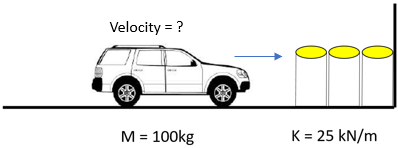## Water Barrels

A car in the shown diagram collides into the water barrels typically found on highways. The barrels act as a spring to reduce impact and deflect 2 meters. If the car weighs 100 kg, and the barrels have a spring constant of 25 kN/m, what is the velocity (m/s) of the car right before impact with the wall?Hint
The spring’s potential energy:
$$U=\frac{kx^{2}}{2}$$$where $$k$$ is the spring constant, and $$x$$ is the compressed distance. Hint 2 The car’s kinetic energy: $$KE=\frac{1}{2}mv^2$$$
where $$m$$ is the mass, and $$v$$ is the velocity.
The spring's potential energy:
$$U=\frac{kx^{2}}{2}=\frac{25kN\cdot (2m)^2}{m\cdot 2}=50kN\cdot m=50,000\:N\cdot m$$$where $$k$$ is the spring constant, and $$x$$ is the compressed distance. The car's kinetic energy: $$KE=\frac{1}{2}mv^2=\frac{1}{2}(100kg)v^2=50kg\cdot v^2$$$
where $$m$$ is the mass, and $$v$$ is the velocity. Because energy is conserved, $$U=KE$$ :
$$50kg\cdot v^2=50,000N\cdot m$$$$$v=31.62\:m/s$$$
31.62 m/s